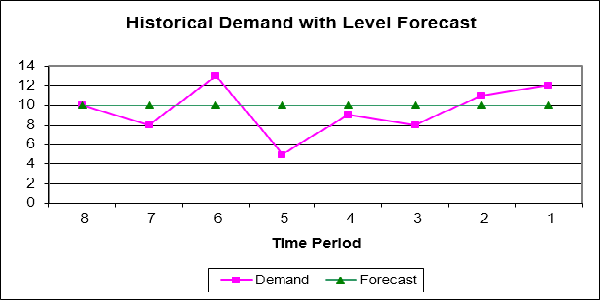# Measuring Supply Chain Forecast AccuracySupply Chain Forecast Accuracy is usually measured with Mean Absolute Percent Error or MAPE, the average of percentage errors. But there are several other metrics to consider. Here’s an example …n = 8 periods
|x| = absolute unsigned value
^2 = squared

Average Error (AE) = (sum (Et))/n = -4/8 = -0.50
Mean Absolute Deviation (MAD) = (sum |Et|)/n = 16/8 = 2.00
Mean Square Error (MSE) = (sum (Et*Et))/n = 48/8 = 6.00
Standard Deviation (SD) = square root((sum(Et*Et))/(n-1)) = sqrt(48/7) = 2.62
Mean Absolute Percent Error (MAPE) = (sum(|Et|/Dt)*100 = 210/8 = 26.25%
Cumulative Forecast Error = sum Et = 16
Cumulative Forecast Error Ratio = (sum |Et|)/(average Ft)
Group Forecast Accuracy = aggregate forecast vs actual